Calculation with Heat Transfer

Calculations of Heat Transfer

Conservation of energy theorem is also applied to heat transfer. In an isolated system, given heat is always equal to taken heat or heat change in the system is equal to zero. If two objects having different temperatures are in contact, heat transfer starts between them. The amount of heat given is equal to the amount of heat taken. Object one has mass m1, temperature t1 and specific heat capacity c1, object two has mass m2, temperature t2 and specific heat capacity c2.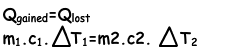Example: Find the final temperature of the mixture, if two cup of water having masses m1=150g and m2=250g and temperatures T1= 30 ºC and T2=75 ºCare mixed in an isolated system in which there is no heat lost. (cwater=1cal/g.ºC)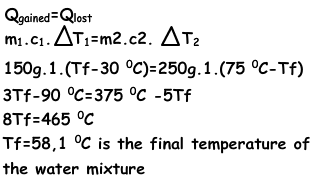Example: Temperature of the iron block decreases from 85 ºC to 25 ºC. If the mass of the block is 1,2kg, calculate the heat lost by the block. (ciron=0.115cal/g.ºC)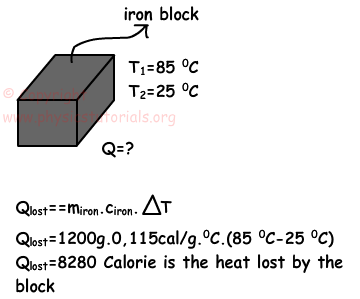Example: The graph given below shows the relation between given heat and change in the temperatures of the three matters having same masses. Compare the specific heat capacities of these matters.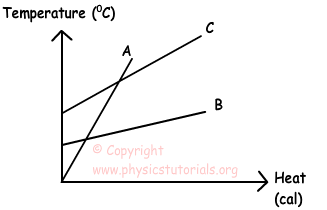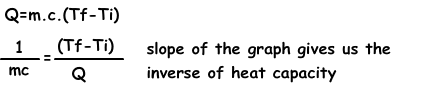Since the masses of these matters are equal, B has the greatest specific heat capacity because, with the same amount of heat, change in the temperature of the B is lower than the other two matters. Moreover, A has the minimum specific heat capacity, because the change in its temperature with the same amount of heat is larger than the others. Finally, specific heat capacity of the C is between A and B. Thus;

cB>cC>cA

Heat Temperature and Thermal Expansion Exams and Solutions

Author:

Tags: